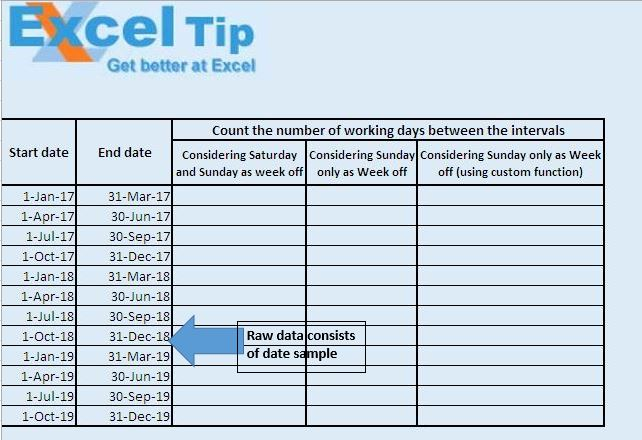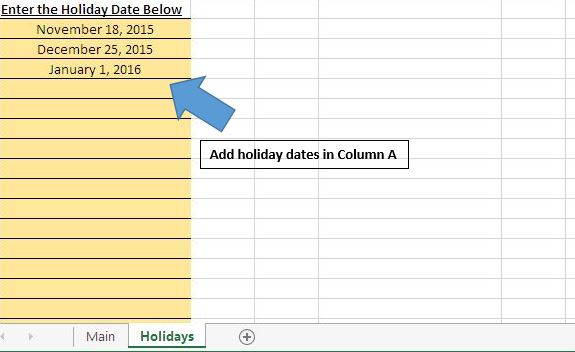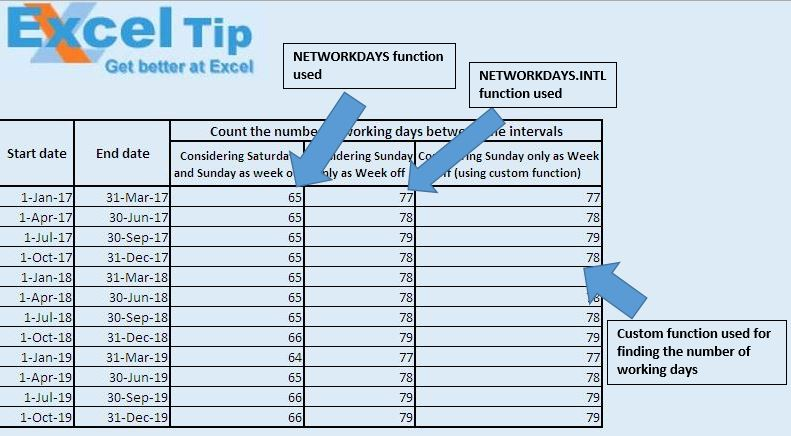# Calculate the count of workdays between two dates using VBA in Microsoft Excel

In this article, we will create a User Defined Function (UDF) to count the number of working days between the specified dates, including or excluding Saturdays and Sundays as week offs.

Raw data for this example consists of a start date and an end date. We want to count the number of working days between these dates.We have specified the holiday dates in the column A in “Holidays” sheet.Excel has inbuilt function, NETWORKDAYS to count the number of working days between the interval.

Syntax of NETWORKDAYS function

NETWORKDAYS(StartDate, EndDate,[Holidays])

This function will exclude the date specified in the Holidays list, while counting the number of working days.

This function considers Saturdays and Sundays as Week offs by default, so we can't count the number of working days, in case we have only one Week off.

We have created “CountWorkingDays” custom function to count the number of working days between the interval. This custom function handles the problem of the NETWORKDAYS function. In this function, we can count the number of working days even if there is only one Week off either on Saturday or Sunday.

Syntax of custom function

CountWorkingDays (StartDate, EndDate, InclSaturdays, InclSundays)

InclSaturdays and InclSundays are optional parameters. By default, both have TRUE values. To change Saturdays and Sundays into working days, change the value of the respective parameter to FALSE.

Microsoft introduced NETWORKDAYS.INTL function with Excel 2010. This function handles the problem of NETWORKDAYS function. In this function, we can specify the Week off days. We can either specify one or two days as Week off.

Syntax of NETWORKDAYS.INTL function

NETWORKDAYS.INTL(StartDate, EndDate, [Weekend], [Holidays])

In the weekend parameter, we can specify Week off days.

In this example, we will use all the above three functions to count the number of working days.Logic explanation

In the “CountWorkingDays” function, firstly we check whether given date in the parameter exists in the specified holiday list. If the date exists in the holiday list, then that day is not counted in the number of working days. If the date doesn’t exists in the holiday list, then check whether given date is Saturday or Sunday. Based on the input parameter provided, check whether to include or exclude Saturdays or Sundays as holiday.

Code explanation

Set RngFind = Worksheets("Holidays").Columns(1).Find(i)

Above code is used to find the location where the specified date exists in the holiday list.

If Not RngFind Is Nothing Then

GoTo ForLast

End If

Above code is used to check whether specified date exists in the holiday list. If condition returns TRUE, then that day is not counted in the number of working days.

```
Option Explicit

Function CountWorkingDays(StartDate As Long, EndDate As Long, Optional InclSaturdays As Boolean = True, _
Optional InclSundays As Boolean = True)

'Declaring variables
Dim RngFind As Range
Dim i As Long

For i = StartDate To EndDate

On Error Resume Next

'Finding the location where the specified date exist in the Holidays sheet
Set RngFind = Worksheets("Holidays").Columns(1).Find(i)

On Error GoTo 0

'Checking whether it is holiday on the given date
If Not RngFind Is Nothing Then
GoTo ForLast
End If

'Checking whether it is Saturday on given date
If InclSaturdays Then
If Weekday(i, 2) = 6 Then
GoTo ForLast
End If
End If

'Checking whether it is Sunday on given date
If InclSundays Then
If Weekday(i, 2) = 7 Then
GoTo ForLast
End If
End If

CountWorkingDays = CountWorkingDays + 1

ForLast:

Next

End Function

```

We would love to hear from you, do let us know how we can improve our work and make it better for you. Write to us at info@exceltip.com

1.hi,
i need a code in vba to find those employee who worked 7 days in a week can you help me.

format of data like that
name, employee id , process , 11/01/2015, to 11/22/2015
akash 57575 tata present ,,,,,,, weekoff, present

•Hi Akash,

We have worked on your requirement..

As you stated that you have data from column A to column Y where first 3 columns that contain "Emp_Name", "Employee ID" & "Process" and rest columns are having attendance. The following code will help you to get the summary of employees in new sheet where you can find employees who worked 7 days in a week as highlighted cells.

```Option Explicit

Sub Calculate_days()

Dim lastcol, lastrow As Long
Dim i, j, k As Long
Dim present As String

lastcol = Range("A1").SpecialCells(xlCellTypeLastCell).Column
lastrow = Range("A1").SpecialCells(xlCellTypeLastCell).Row
present = "P"

Sheets("Sheet1").Copy after:=Sheets(Sheets.Count)

Range("A1").Select
Cells.Copy
Selection.PasteSpecial Paste:=xlPasteValues

ActiveSheet.Name = "Summary"

For j = 2 To lastrow
For i = 4 To lastcol
If Weekday(Cells(1, i).Value, 2) = 1 Then
Cells(j, i) = Application.WorksheetFunction.CountIf(Range(Cells(j, i), Cells(j, i + 6)), present)

End If
Next i
Next j

For j = 2 To lastrow
For i = 4 To 10
If Weekday(Cells(1, i).Value, 2) = 1 And i > 4 Then
Cells(j, i - 1) = Application.WorksheetFunction.CountIf(Range(Cells(j, 4), Cells(j, i - 1)), present)

Exit For
End If
Next i

Next j

Cells(1, i - 1).Value = "11/2/2015"

For i = lastcol To 4 Step -1
If Weekday(Cells(1, i).Value, 2) <> 1 Then
Columns(i).Delete
End If
Next i

ActiveWorkbook.Save

lastcol = Range("A1").SpecialCells(xlCellTypeLastCell).Column
lastrow = Range("A1").SpecialCells(xlCellTypeLastCell).Row

k = 0
For i = 4 To lastcol
k = k + 1
Cells(1, i).Value = "Week - " & k
Next

For j = 2 To lastrow
For i = 4 To lastcol
If Cells(j, i) = 7 Then
Cells(j, i).Select
Selection.Font.Bold = True
With Selection.Font
.Color = -16776961
End With
With Selection.Interior
.Pattern = xlSolid
.PatternColorIndex = xlAutomatic
.Color = 65535
End With
End If
Next i
Next j

Range("A1").Select

End Sub
```

Happy Learning,
2.